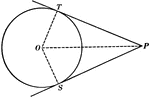### Equal Tangents to Circle Theorem

Illustration used to show that "If two tangents are drawn from any given point to a circle, those tangents…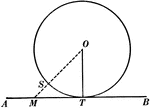### Tangent to Perpendicular Radius Circle Theorem

Illustration used to show that "A tangent to a circle is perpendicular to the radius drawn to the point…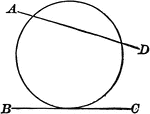### Circle with Secant and Point of Tangency

Illustrations of a circle with secant AD and line BC tangent to it.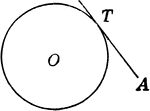### Point of Tangency

Illustration of point of tangency (line and circle).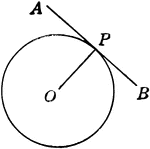### Point of Tangency

Illustration of radius drawn to point of contact of a tangent.